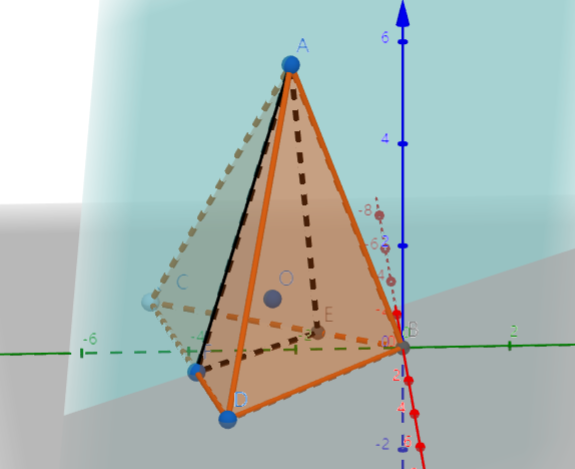# SAT1000 - P594

Geometry Level 3As shown above, in triangular pyramid $A-BCD$, the cross section $AEF$ passes through the center of the inscribed sphere $O$ (i.e. The sphere which is tangent to all of the faces of the solid) of $A-BCD$, and it intersects with $BC, DC$ at point $E,F$ respectively.

If the cross section divides the pyramid into two parts whose volumes are equal, $S_1$ is the surface area of solid $A-BEFD$, $S_2$ is the surface area of solid $A-EFC$, what is always true for $S_1$ and $S_2$?

Have a look at my problem set: SAT 1000 problems

×